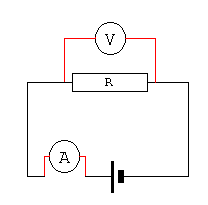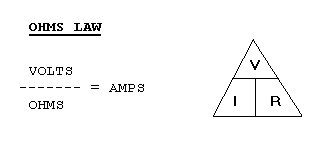Home > Electronics Tutorials > Direct Current (DC) Theory > Ohm's Law Tutorial

Direct Current (DC) Theory - Electronics Teaching

# What is Ohm's Law? - Ohm's Law TutorialThe voltmeter is connected across the resistor, to measure the voltage across the resistor. The ammeter is connected in series with the resistor, to measure the current flowing around the circuit and through the resistor.

Mr Ohm discovered that if you double the voltage across the resistor then the current through it doubles. If you halve the voltage then the current is halved. This means that the current is PROPORTIONAL to the voltage.

He also found that if you double the value of the resistor then the current through it is halved. If the value of the resistor is halved the the current is doubled. Thus the current is INVERSELY PROPORTIONAL to the resistance.To use the VIR triangle place your finger over the value you wish to find. If you wish to find V then multiply I by R. If you wish to find I then divide V by R.